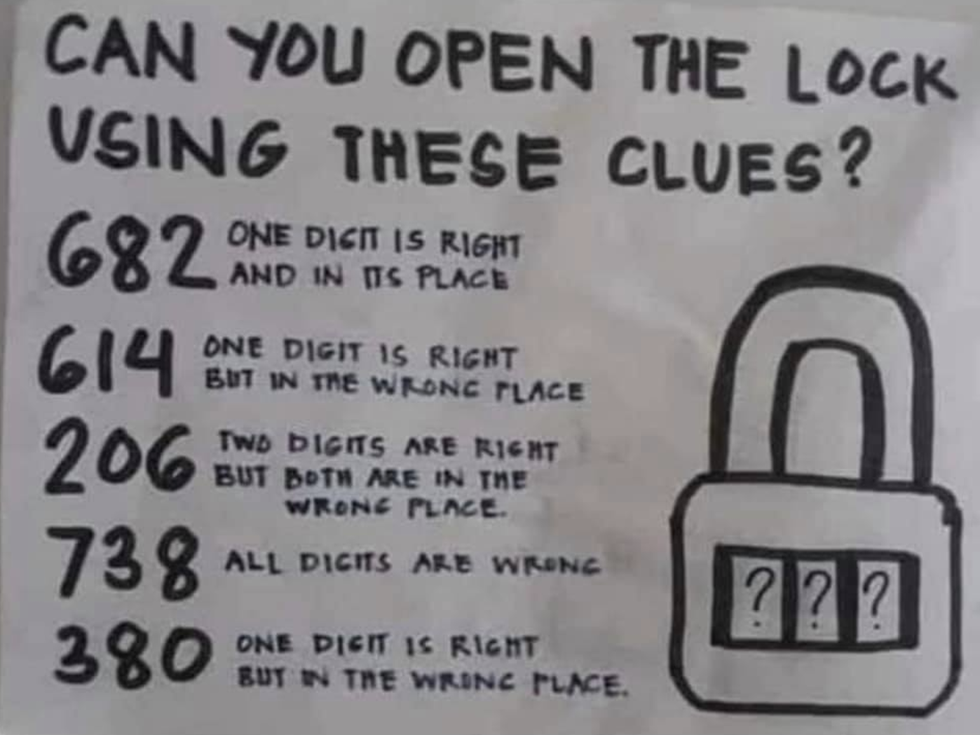# Clojure Core Logic for a PuzzleI saw a puzzle pop up on Facebook a couple weeks ago, and it looked like a fun exercise for core.logic, since the puzzle simply requires keeping track of some constraints and reconciling them to one answer.

I had previously tinkered with the core.logic primer and I referred back to it to complete this little puzzle. I had originally coded some more complete rules about exclusion of some values which could have been implied by the puzzle, but I found they could be dropped and still get down to one answer. I started from the entire problem space of all digits and added the constraints to watch and verify each constraint’s effects.

```(ns scratch.2020-05
(:require [clojure.core.logic :as l]))

(l/run* [a b c]
;; all digits 0-9
(l/membero a (range 10))
(l/membero b (range 10))
(l/membero c (range 10))
;; 6 8 2 one digit is right and in its right place
(l/conde
[(l/== a 6)]
[(l/== b 8)]
[(l/== c 2)])
;; 6 1 4 one digit is right but in the wrong place
(l/conde
[(l/membero a [1 4])]
[(l/membero b [6 4])]
[(l/membero c [6 1])])
;; 206 2 digits are right, but both are in the wrong place
(l/conde
[(l/membero a [0 6])
(l/membero b [2 6])
(l/membero c (remove #{2 0 6} (range 10)))]
[(l/membero a [0 6])
(l/membero c [2 0])
(l/membero b (remove #{2 0 6} (range 10)))]
[(l/membero b [2 6])
(l/membero c [2 0])
(l/membero a (remove #{2 0 6} (range 10)))]
)
;; 3 8 0 one digit is right but in the wrong place
(l/conde
[(l/membero a [8 0])]
[(l/membero b [3 0])]
[(l/membero c [3 8])])
;; 7 3 8 all the digits are wrong
(l/membero a (remove #{7 3 8} (range 10)))
(l/membero b (remove #{7 3 8} (range 10)))
(l/membero c (remove #{7 3 8} (range 10))))
;; => ([0 4 2])```

Filed Under: Code Clojure